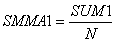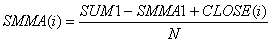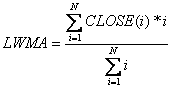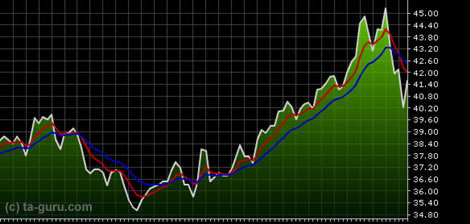Chart Pro online
User name (email):

 Recently used

 Forex

 Indices

# Moving average

Moving average is perhaps the most used technical indicator in technical analysis. It belongs to the category of leading indicators. With its visibility it makes easier to identify trends.

What is moving average? As it could be concluded by its name, it is the average of certain data. For example, moving average can be created based on the average closing price over the past ten days. All closing prices in the last 10 days are added, and the sum is divided by 10 (creating the average for the period). Then, for every next day ten-day average is created - new day is included in average and the first is removed. Therefore, it is called "moving" average.

Moving average line softens price movements, so it is easier to identify trend. The indicator can be used to identify trends, but also to detect the moments of trend reversal.Moving average

There are different types of moving averages:

• Simple moving average (SMA)• Exponential moving average (EMA)
EMA (i) = (CLOSE (i) * P) + EMA (i-1) * (1-P)
- CLOSE (i): closing price for the current period
- P: percentage of price use
- EMA (i-1): exponential moving average for the previous period. EMA (0) = SMA.
• Smoothed moving average (SMMA)• Linear weighted moving average (LWMA)• Triangular moving average (TMA)
TMA = SMA of SMA

All these moving averages are using the closing price, but not only the closing price could be used as the calculation parameter. Open, high and low could also be used. Some even take as parameter the median price ((high + low) / 2), but the most common is use of closing price.SMA example

Interpretation

Buying and selling signals can be determined using one moving average: selling signal is generated when closing price crosses below MA. If closing price crosses above MA, buying signal is generated. In case of smooth line intersections false signals could be generated. Therefore, during the interpretation of moving average we should stick to certain rules:

(1) If the moving average line settles down or progress after the fall and closing price crosses above MA, strong buying signal is generated.

(2) If closing price crosses below the moving average and moving average continues to rise further, buying signal is generated.

(3) If closing price is above MA and falls towards it, if price touches moving average and rises after that, buying signal is created.

(4) If the closing price drops far below the falling MA, it can be expected that it will for at least short period of time rise towards the MA, so buying signal is created.

Selling signals

(1) If the moving average line settle down or falls after rising and closing price crosses below MA, strong selling signal is generated.

(2) If closing price crosses above the moving average and moving average continues to fall further, selling signal is generated.

(3) If closing price is below MA and rises towards it, if price touches moving average and declines after that, selling signal is created.

(4) If the closing price rises far above the growing MA, it can be expected that it will for at least short period of time fall towards the MA, so selling signal is created.

Although buying and selling signals could be generated using one moving average, traders usually calculate signals using two moving averages with different base. If shorter moving average (e.g. SMA (5)) crosses above the longer moving average (e.g. SMA (20)), buying signal is generated. If opposite is true, selling signal is generated.Two moving averages

Signals can also be generated on the basis of three moving averages. The most popular system is 4-9-18. In an upward trend, layout of moving averages should be: 4-day average is above the 9-day average, and 9-day average is above the 18-day average. The downward trend has reversed layout. When 4-day average crosses above the 9-day and 18-day average in the downward trend, buying warning is generated. Buying signal must be confirmed with 9-day average, it should cross above 18-day average. When 4-day average crosses below 9-day and 18-day average in an upward trend, selling warning is generated. Selling signal must also be confirmed by 9-day average - it should cross below the 18-day average.

Example